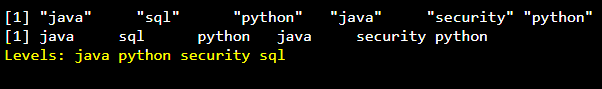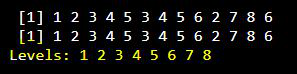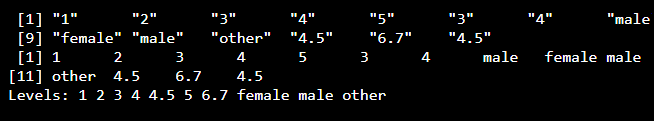Open in App
Not now

# Find the levels of factor of a given vector in R

• Last Updated : 23 May, 2021

Factors are the objects which are used to categorize the data and display the data as levels. The objects can be integer, character. They can be used to find the unique values in the given vector. The resulting data is known as levels. Factors are useful in statistical analysis in analyzing the categorical data. It is used to find the levels of the given vector. In this article, we are going to find the levels of factor in the given vector in R.

Example:

Input:

data=[female,female,male,male,other]

Output:

factor=female,male,other.

We can convert the vector data into factor by using factor() function, with this we can get levels() of the given vector

Syntax:

factor(vector_name)

Result is factor levels.

Example:

## R

 `# create a vector with subjects data``data=``c``(``"java"``,``"sql"``,``"python"``,``"java"``,``       ``"security"``,``"python"``)`` ` `print``(data)`` ` `# apply factor``a=``factor``(data)`` ` `print``(a)`

Output:Example2:

## R

 `# create a vector with numeric data``data=``c``(1,2,3,4,5,3,4,5,6,2,7,8,6)`` ` `print``(data)`` ` `# apply factor``a=``factor``(data)`` ` `print``(a)`

Output:Example 3:

## R

 `# create a vector with all type of data``data=``c``(1,2,3,4,5,3,4,``"male"``,``"female"``,``"male"``,``       ``"other"``,4.5,6.7,4.5)`` ` `print``(data)`` ` `# apply factor``a=``factor``(data)`` ` `print``(a)`

Output:My Personal Notes arrow_drop_up